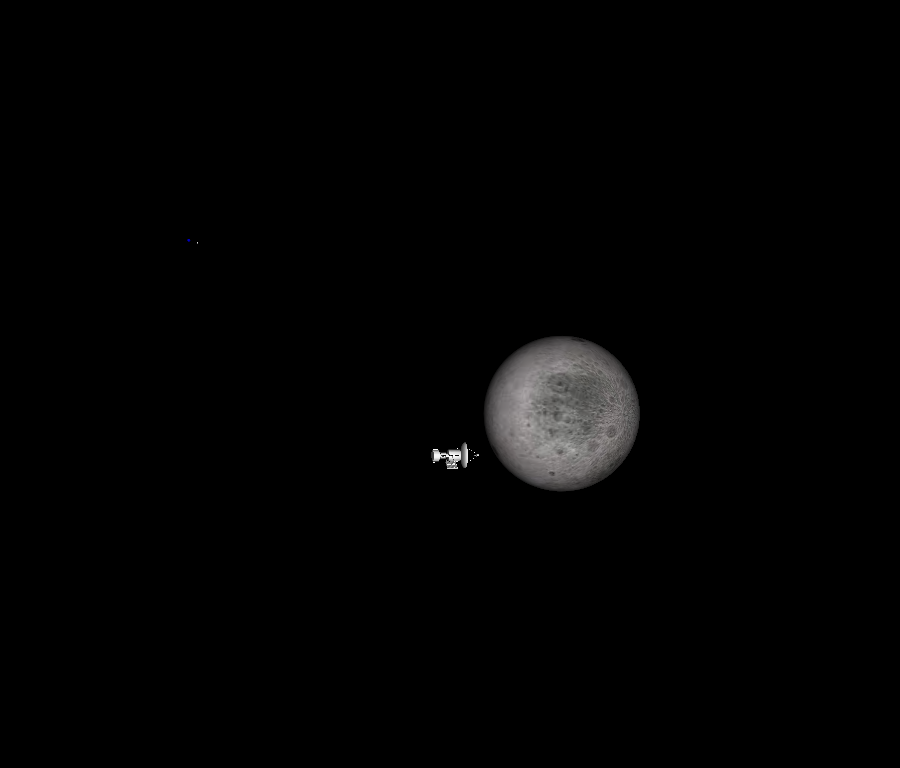# Texture Sphere Animation#

In this tutorial, we will show how to animate a textured sphere.

```import itertools

import numpy as np

from fury import actor, io, utils, window
from fury.data import (
fetch_viz_models,
fetch_viz_textures,
)
```

Create a scene to start.

```scene = window.Scene()
```

Next, load in a texture for each of the actors. For this tutorial, we will be creating one textured sphere for the Earth, and another for the moon. Collect the Earth texture from the FURY github using `fetch_viz_textures` and `read_viz_textures`, then use `io.load_image` to load in the image.

```fetch_viz_textures()
```
```Dataset is already in place. If you want to fetch it again please first remove the folder /Users/skoudoro/.fury/textures
```

Using `actor.texture_on_sphere()`, create an earth_actor with your newly loaded texture.

```earth_actor = actor.texture_on_sphere(earth_image)
```

Then, do the same for the moon.

```moon_filename = read_viz_textures('moon-8k.jpg')

moon_actor = actor.texture_on_sphere(moon_image)
```

```scene.add(earth_actor)
```

Next, alter the position and scale of the moon to correctly size it in comparison to the Earth using `actor.SetPosition()` and `actor.SetScale()`, and rotate the Earth using `utils.rotate` to correctly align the texture.

```moon_actor.SetPosition(1, 0.1, 0.5)
moon_actor.SetScale(0.25, 0.25, 0.25)
utils.rotate(earth_actor, (-90, 1, 0, 0))
```

The ShowManager class is the interface between the scene, the window and the interactor.

```showm = window.ShowManager(
scene, size=(900, 768), reset_camera=False, order_transparent=True
)
```

Next, let’s focus on creating the animation. We can determine the duration of animation with using the `counter`. Use itertools to avoid global variables.

```counter = itertools.count()
```

Use `set_camera` to ensure the camera is in the optimal position for the scene.

```scene.set_camera(
position=(0.24, 0.00, 4.34),
focal_point=(0.00, 0.00, 0.00),
view_up=(0.00, 1.00, 0.00),
)
```

Let’s create a sphere actor to add to the Earth. We will place this sphere on the Earth’s surface on Bloomington, IN, home of FURY’s headquarters!

```center = np.array([[-0.39, 0.3175, 0.025]])
```

Also creating a text actor to add below the sphere.

```text_actor = actor.text_3d(
'Bloomington, Indiana', (-0.42, 0.31, 0.03), window.colors.white, 0.004
)
utils.rotate(text_actor, (-90, 0, 1, 0))
```

Let’s also import a model of a satellite to visualize circling the moon.

```fetch_viz_models()
satellite_actor = utils.get_actor_from_polydata(satellite)

satellite_actor.SetPosition(-0.75, 0.1, 0.4)
satellite_actor.SetScale(0.005, 0.005, 0.005)
```
```Dataset is already in place. If you want to fetch it again please first remove the folder /Users/skoudoro/.fury/models
```

In the `timer_callback` function, use if statements to specify when certain events will happen in the animation, based on the position that the counter is at. So, for example, the earth actor will continue to rotate while the count is less than 450.

```def timer_callback(_obj, _event):
cnt = next(counter)
showm.render()
if cnt < 450:
utils.rotate(earth_actor, (1, 0, 1, 0))
if cnt % 5 == 0 and cnt < 450:
showm.scene.azimuth(-1)
if cnt == 300:
scene.set_camera(
position=(-3.679, 0.00, 2.314),
focal_point=(0.0, 0.35, 0.00),
view_up=(0.00, 1.00, 0.00),
)
if cnt > 300 and cnt < 450:
scene.zoom(1.01)
if cnt >= 450 and cnt < 1500:
if cnt >= 450 and cnt < 550:
scene.zoom(1.01)
if cnt == 575:
moon_actor.SetPosition(-1, 0.1, 0.5)
scene.set_camera(
position=(-0.5, 0.1, 0.00),
focal_point=(-1, 0.1, 0.5),
view_up=(0.00, 1.00, 0.00),
)
scene.zoom(0.03)
utils.rotate(satellite_actor, (180, 0, 1, 0))
scene.rm(earth_actor)
if cnt > 575 and cnt < 750:
showm.scene.azimuth(-2)
utils.rotate(moon_actor, (-2, 0, 1, 0))
satellite_actor.SetPosition(-0.8, 0.1 - cnt / 10000, 0.4)
if cnt >= 750 and cnt < 1100:
showm.scene.azimuth(-2)
utils.rotate(moon_actor, (-2, 0, 1, 0))
satellite_actor.SetPosition(-0.8, -0.07 + cnt / 10000, 0.4)
if cnt == 1100:
showm.exit()
```

Watch your new animation take place!

```showm.add_timer_callback(True, 35, timer_callback)
showm.start()
window.record(showm.scene, size=(900, 768), out_path='viz_earth_animation.png')
```Total running time of the script: ( 0 minutes 40.178 seconds)

Gallery generated by Sphinx-Gallery